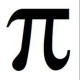# Dudeney numbers5.00 (1 votes)Loading...

This is pretty neat. A Dudeney number is a positive integer that is a perfect cube such that the sum of its decimal digits is equal to the cube root of the number. For example:

5832 = 18 x 18 x 18… and 5 + 8 + 3 + 2 = 18.

How many such numbers do you think exist?

Well, it turns out there is exactly 6. They are: 1, 512, 4913, 5832, 17576 and 19683.

### 2 thoughts on “Dudeney numbers”

1.Hey, two of them are powers of 2 😛

2.Just a note:
1 = 1 x 1 x 1 and 1 = 1
512 = 8 x 8 x 8 and 8 = 5 + 1 + 2
4913 = 17 x 17 x 17 and 17 = 4 + 9 + 1 + 3
5832 = 18 x 18 x 18 and 18 = 5 + 8 + 3 + 2
17576 = 26 x 26 x 26 and 26 = 1 + 7 + 5 + 7 + 6
19683 = 27 x 27 x 27 and 27 = 1 + 9 + 6 + 8 + 3